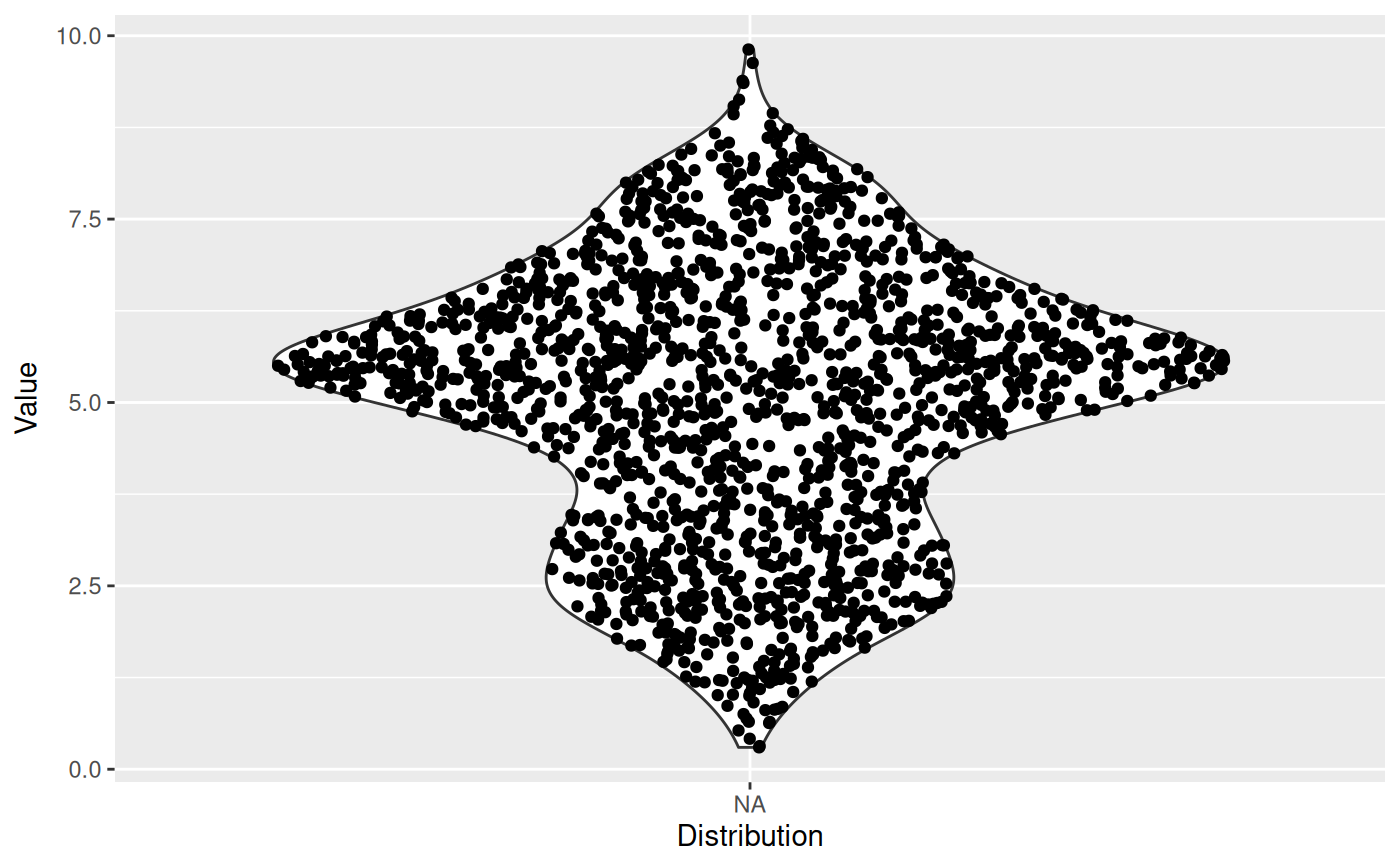The sina plot is a data visualization chart suitable for plotting any single variable in a multiclass dataset. It is an enhanced jitter strip chart, where the width of the jitter is controlled by the density distribution of the data within each class.

stat_sina(
mapping = NULL,
data = NULL,
geom = "point",
position = "dodge",
scale = "area",
method = "density",
bw = "nrd0",
kernel = "gaussian",
maxwidth = NULL,
bin_limit = 1,
binwidth = NULL,
bins = NULL,
seed = NA,
jitter_y = TRUE,
...,
na.rm = FALSE,
orientation = NA,
show.legend = NA,
inherit.aes = TRUE
)

geom_sina(
mapping = NULL,
data = NULL,
stat = "sina",
position = "dodge",
...,
na.rm = FALSE,
orientation = NA,
show.legend = NA,
inherit.aes = TRUE
)

## Arguments

mapping

Set of aesthetic mappings created by aes(). If specified and inherit.aes = TRUE (the default), it is combined with the default mapping at the top level of the plot. You must supply mapping if there is no plot mapping.

data

The data to be displayed in this layer. There are three options:

If NULL, the default, the data is inherited from the plot data as specified in the call to ggplot().

A data.frame, or other object, will override the plot data. All objects will be fortified to produce a data frame. See fortify() for which variables will be created.

A function will be called with a single argument, the plot data. The return value must be a data.frame, and will be used as the layer data. A function can be created from a formula (e.g. ~ head(.x, 10)).

geom

The geometric object to use to display the data, either as a ggproto Geom subclass or as a string naming the geom stripped of the geom_ prefix (e.g. "point" rather than "geom_point")

position

Position adjustment, either as a string naming the adjustment (e.g. "jitter" to use position_jitter), or the result of a call to a position adjustment function. Use the latter if you need to change the settings of the adjustment.

scale

How should each sina be scaled. Corresponds to the scale parameter in ggplot2::geom_violin()? Available are:

• 'area' for scaling by the largest density/bin among the different sinas

• 'count' as above, but in addition scales by the maximum number of points in the different sinas.

• 'width' Only scale according to the maxwidth parameter

For backwards compatibility it can also be a logical with TRUE meaning area and FALSE meaning width

method

Choose the method to spread the samples within the same bin along the x-axis. Available methods: "density", "counts" (can be abbreviated, e.g. "d"). See Details.

bw

The smoothing bandwidth to be used. If numeric, the standard deviation of the smoothing kernel. If character, a rule to choose the bandwidth, as listed in stats::bw.nrd().

kernel

Kernel. See list of available kernels in density().

maxwidth

Control the maximum width the points can spread into. Values between 0 and 1.

A multiplicate bandwidth adjustment. This makes it possible to adjust the bandwidth while still using the a bandwidth estimator. For example, adjust = 1/2 means use half of the default bandwidth.

bin_limit

If the samples within the same y-axis bin are more than bin_limit, the samples's X coordinates will be adjusted.

binwidth

The width of the bins. The default is to use bins bins that cover the range of the data. You should always override this value, exploring multiple widths to find the best to illustrate the stories in your data.

bins

Number of bins. Overridden by binwidth. Defaults to 50.

seed

A seed to set for the jitter to ensure a reproducible plot

jitter_y

If y is integerish banding can occur and the default is to jitter the values slightly to make them better distributed. Setting jitter_y = FALSE turns off this behaviour

...

Other arguments passed on to layer(). These are often aesthetics, used to set an aesthetic to a fixed value, like colour = "red" or size = 3. They may also be parameters to the paired geom/stat.

na.rm

If FALSE, the default, missing values are removed with a warning. If TRUE, missing values are silently removed.

orientation

The orientation of the layer. The default (NA) automatically determines the orientation from the aesthetic mapping. In the rare event that this fails it can be given explicitly by setting orientation to either "x" or "y". See the Orientation section for more detail.

show.legend

logical. Should this layer be included in the legends? NA, the default, includes if any aesthetics are mapped. FALSE never includes, and TRUE always includes. It can also be a named logical vector to finely select the aesthetics to display.

inherit.aes

If FALSE, overrides the default aesthetics, rather than combining with them. This is most useful for helper functions that define both data and aesthetics and shouldn't inherit behaviour from the default plot specification, e.g. borders().

stat

The statistical transformation to use on the data for this layer, either as a ggproto Geom subclass or as a string naming the stat stripped of the stat_ prefix (e.g. "count" rather than "stat_count")

## Details

There are two available ways to define the x-axis borders for the samples to spread within:

• method == "density"A density kernel is estimated along the y-axis for every sample group, and the samples are spread within that curve. In effect this means that points will be positioned randomly within a violin plot with the same parameters.

• method == "counts":The borders are defined by the number of samples that occupy the same bin.

## Aesthetics

geom_sina understand the following aesthetics (required aesthetics are in bold):

• x

• y

• color

• group

• size

• alpha

## Computed variables

density

The density or sample counts per bin for each point

scaled

density scaled by the maximum density in each group

n

The number of points in the group the point belong to

## Orientation

This geom treats each axis differently and, thus, can thus have two orientations. Often the orientation is easy to deduce from a combination of the given mappings and the types of positional scales in use. Thus, ggplot2 will by default try to guess which orientation the layer should have. Under rare circumstances, the orientation is ambiguous and guessing may fail. In that case the orientation can be specified directly using the orientation parameter, which can be either "x" or "y". The value gives the axis that the geom should run along, "x" being the default orientation you would expect for the geom.

## Author

Nikos Sidiropoulos, Claus Wilke, and Thomas Lin Pedersen

## Examples

ggplot(midwest, aes(state, area)) + geom_point()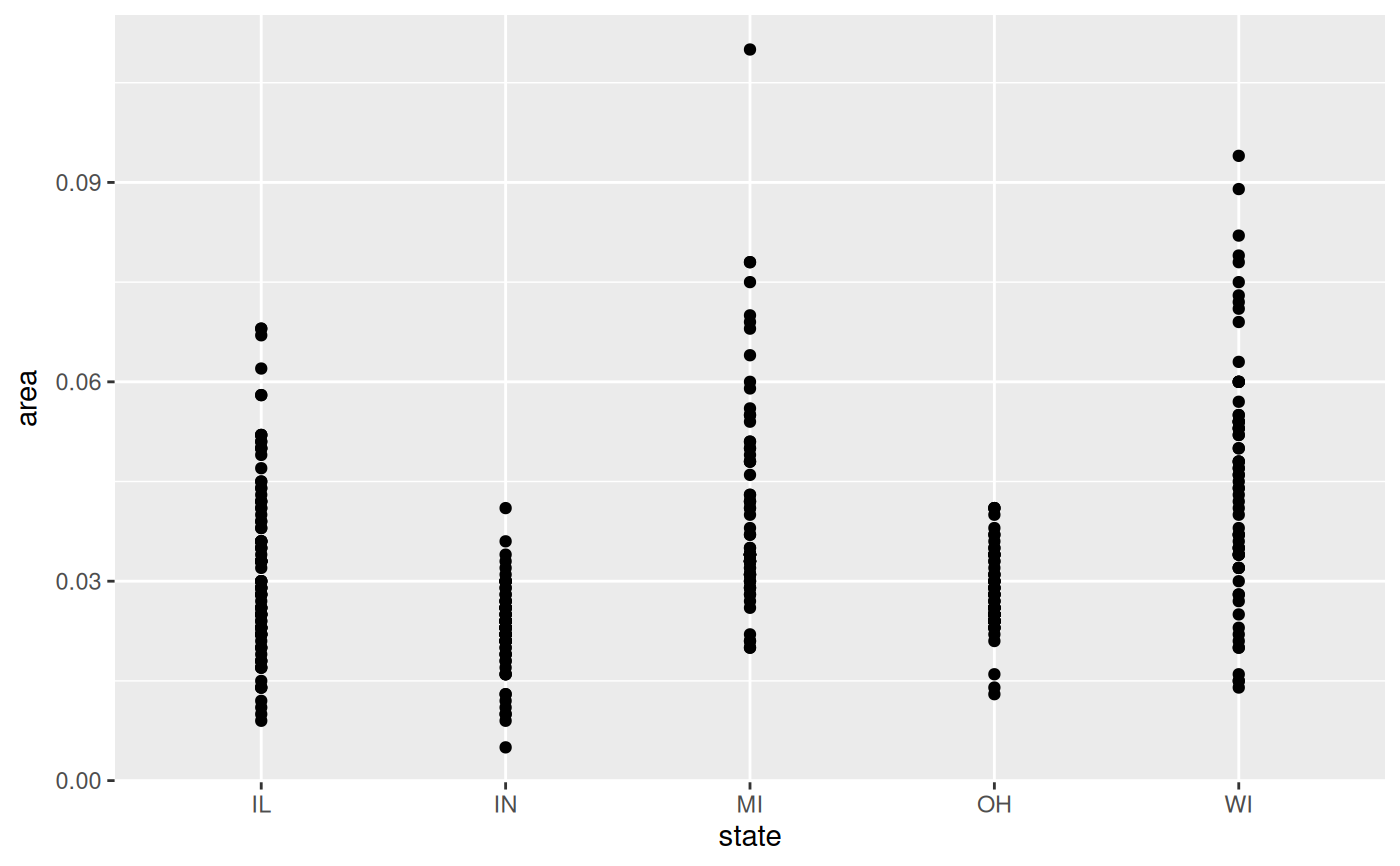# Boxplot and Violin plots convey information on the distribution but not the
# number of samples, while Jitter does the opposite.
ggplot(midwest, aes(state, area)) +
geom_violin()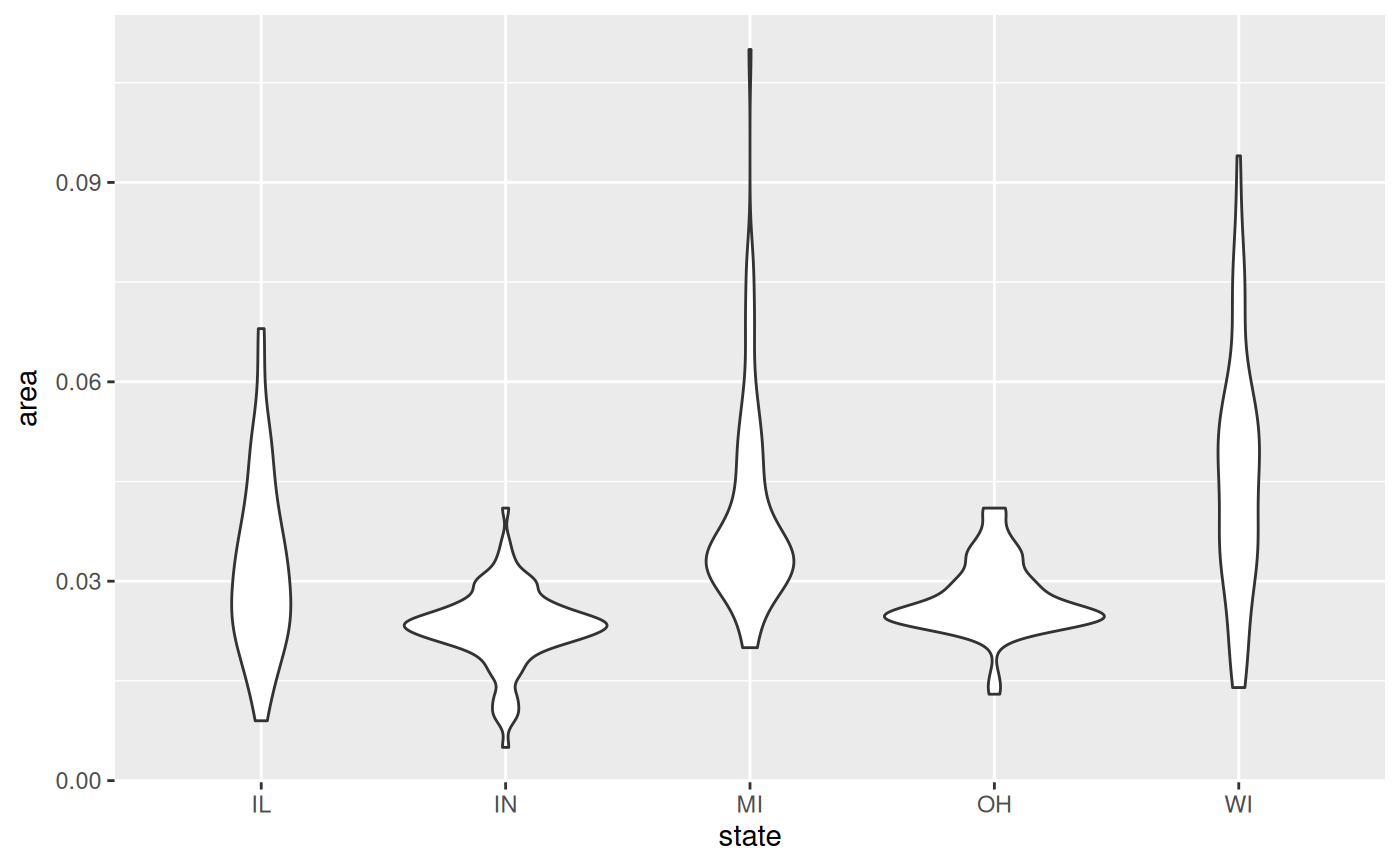ggplot(midwest, aes(state, area)) +
geom_jitter()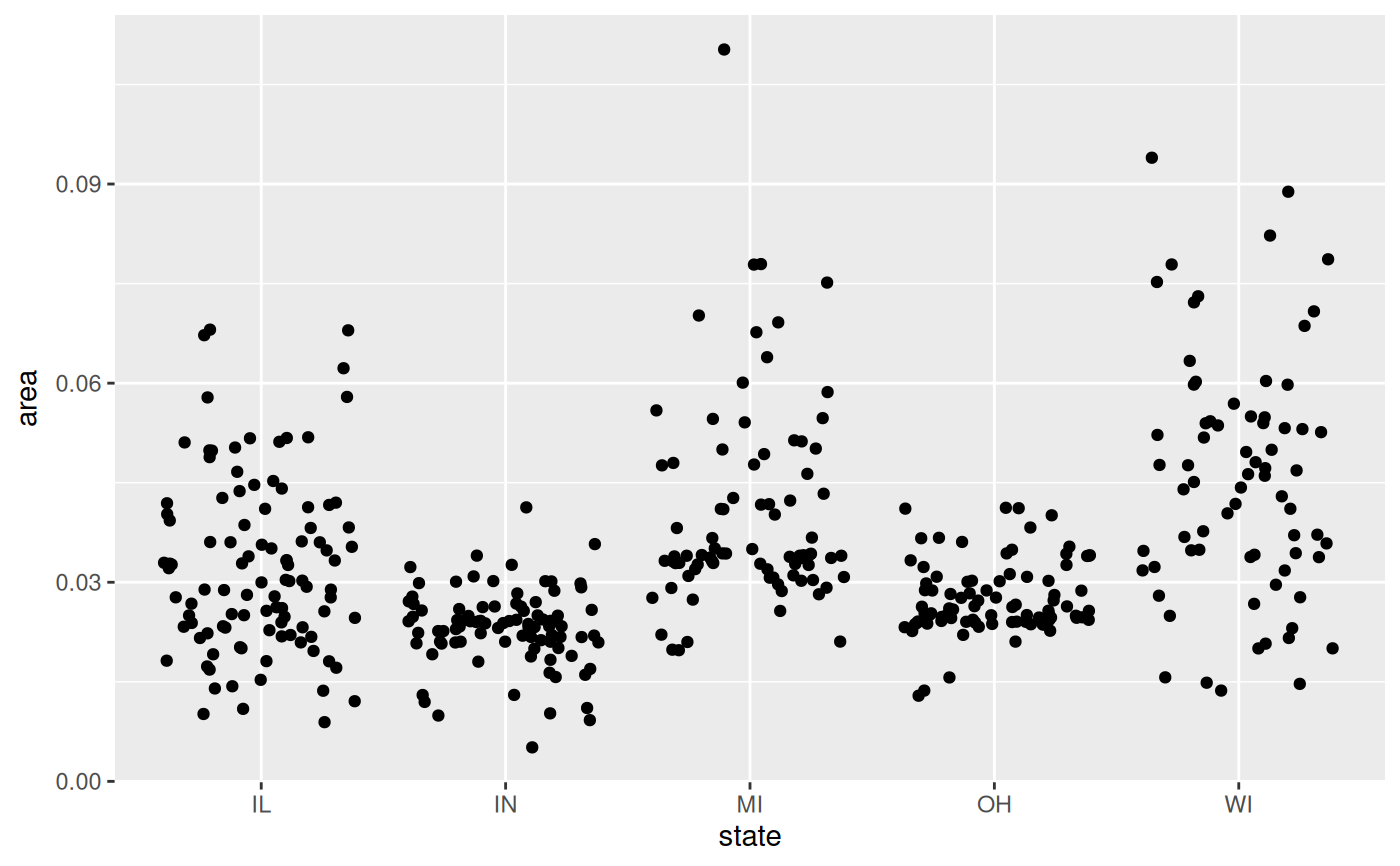# Sina does both!
ggplot(midwest, aes(state, area)) +
geom_violin() +
geom_sina()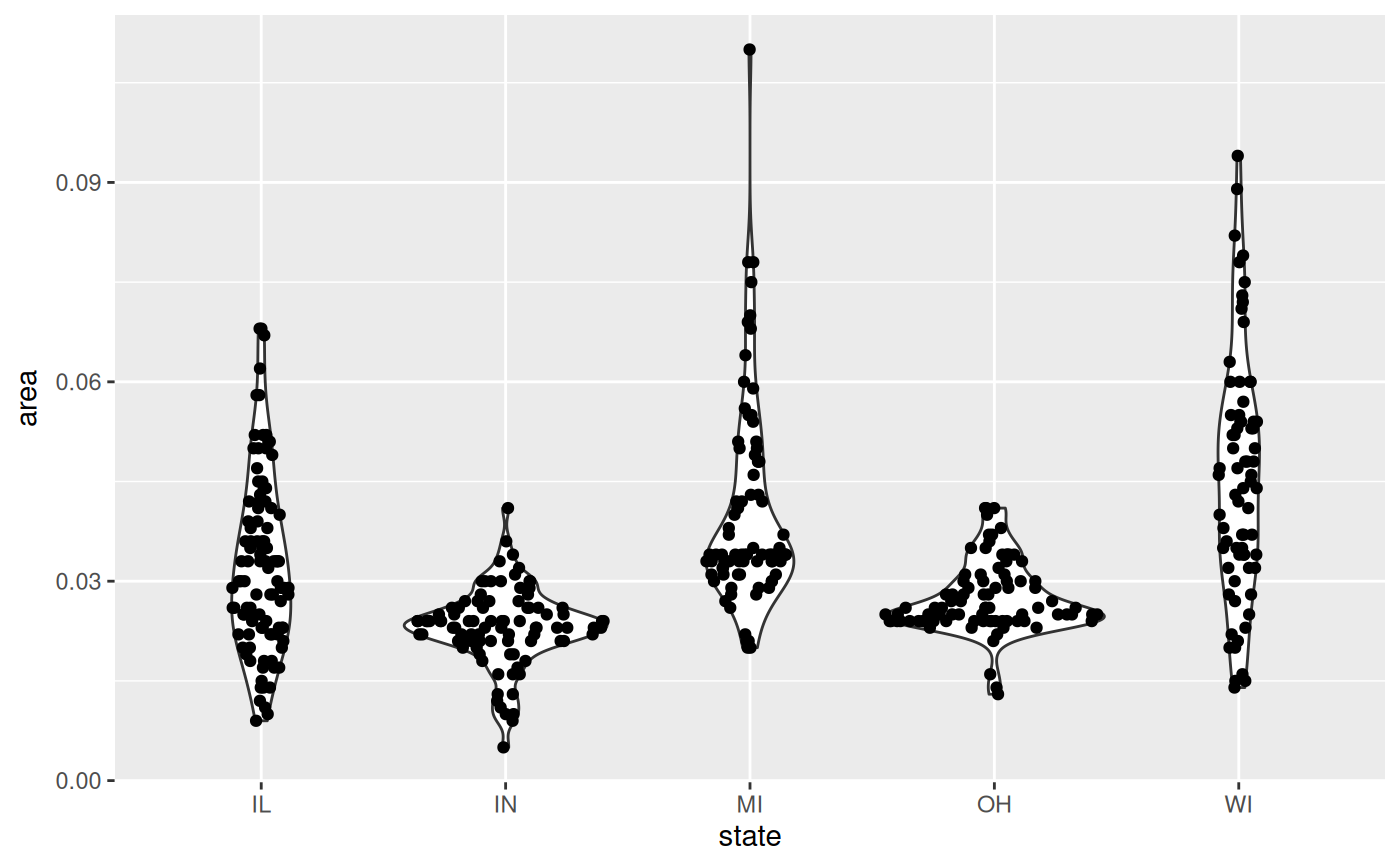p <- ggplot(midwest, aes(state, popdensity)) +
scale_y_log10()

p + geom_sina()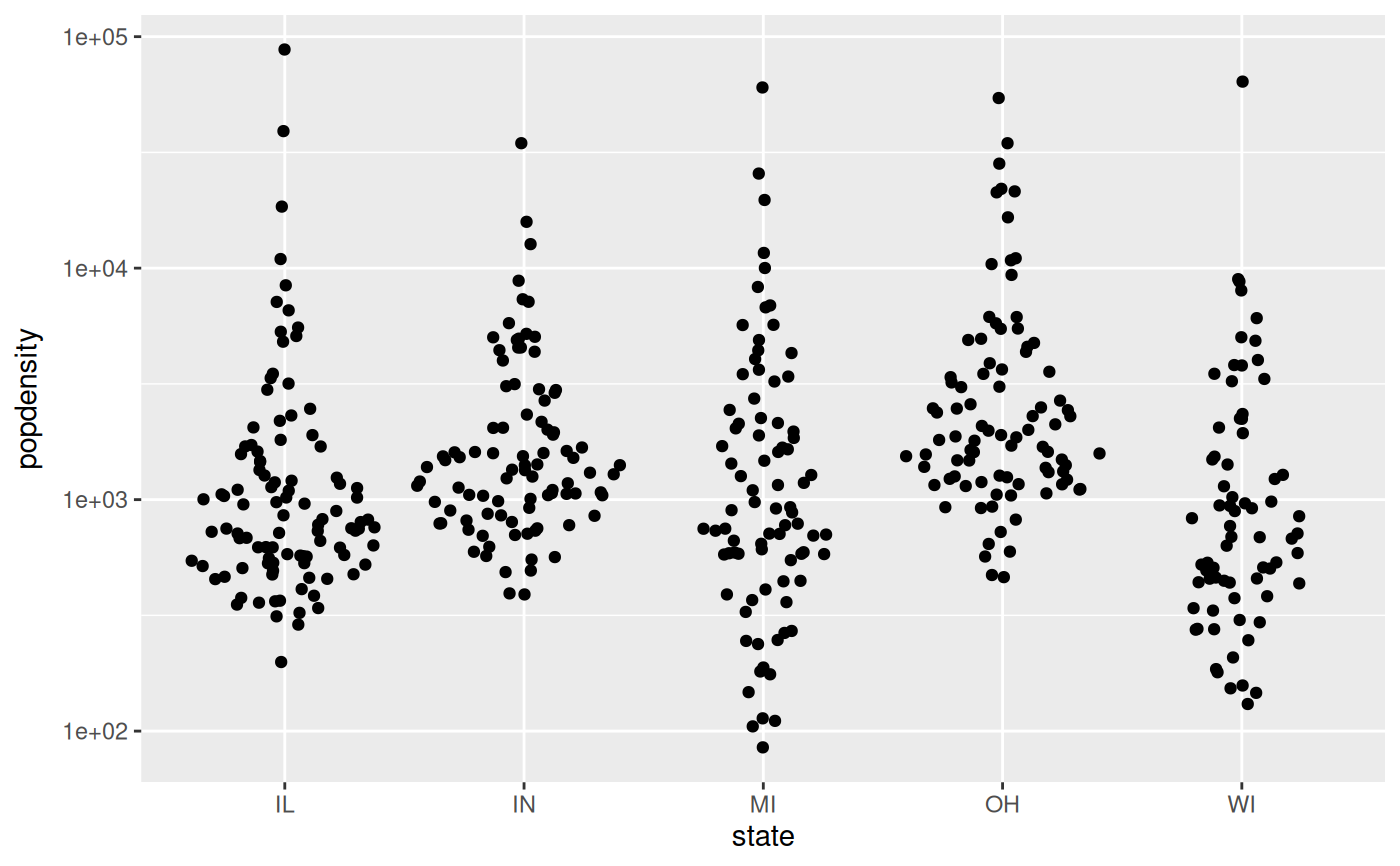# Colour the points based on the data set's columns
p + geom_sina(aes(colour = inmetro))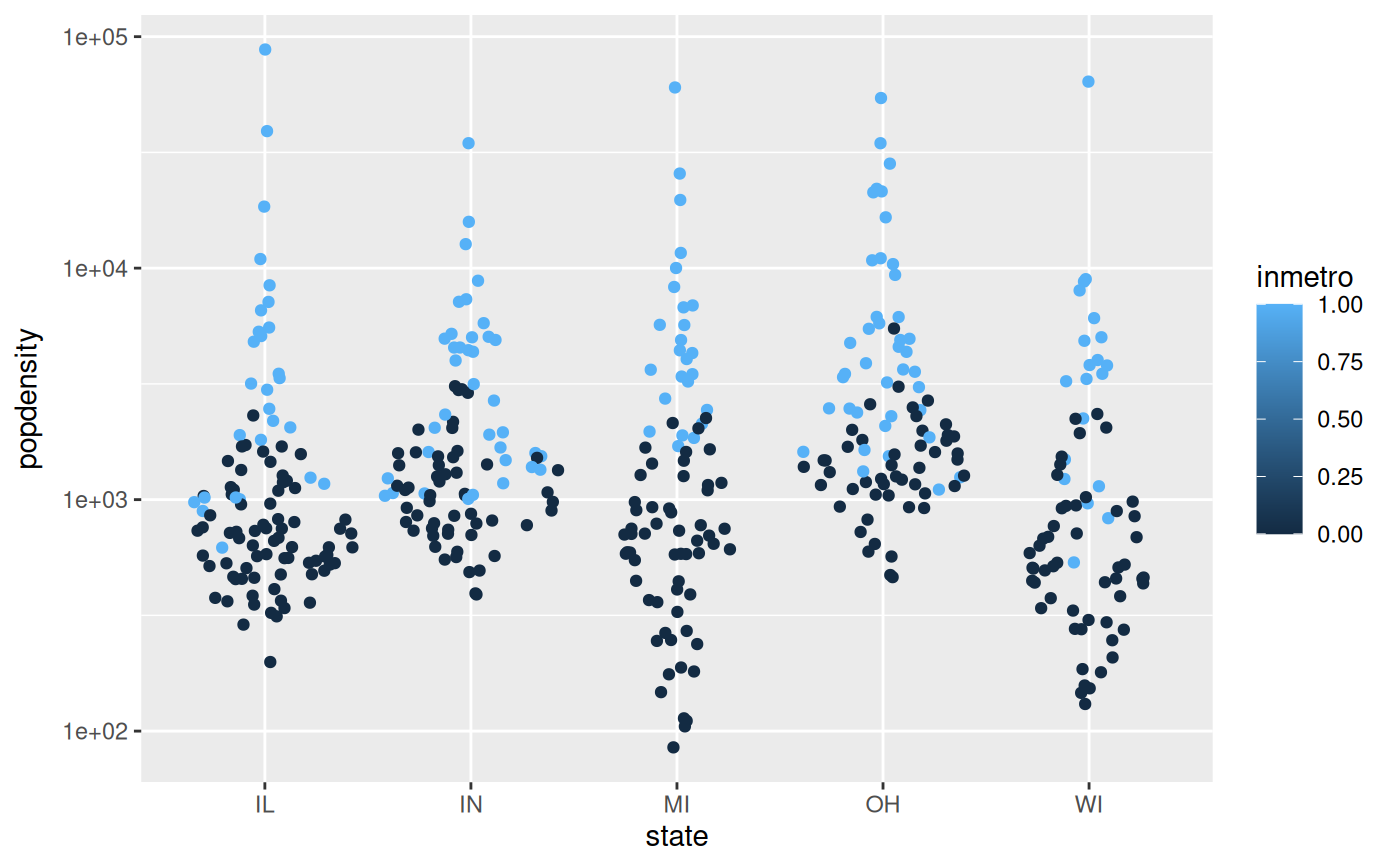# Or any other way
cols <- midwest$popdensity > 10000 p + geom_sina(colour = cols + 1L)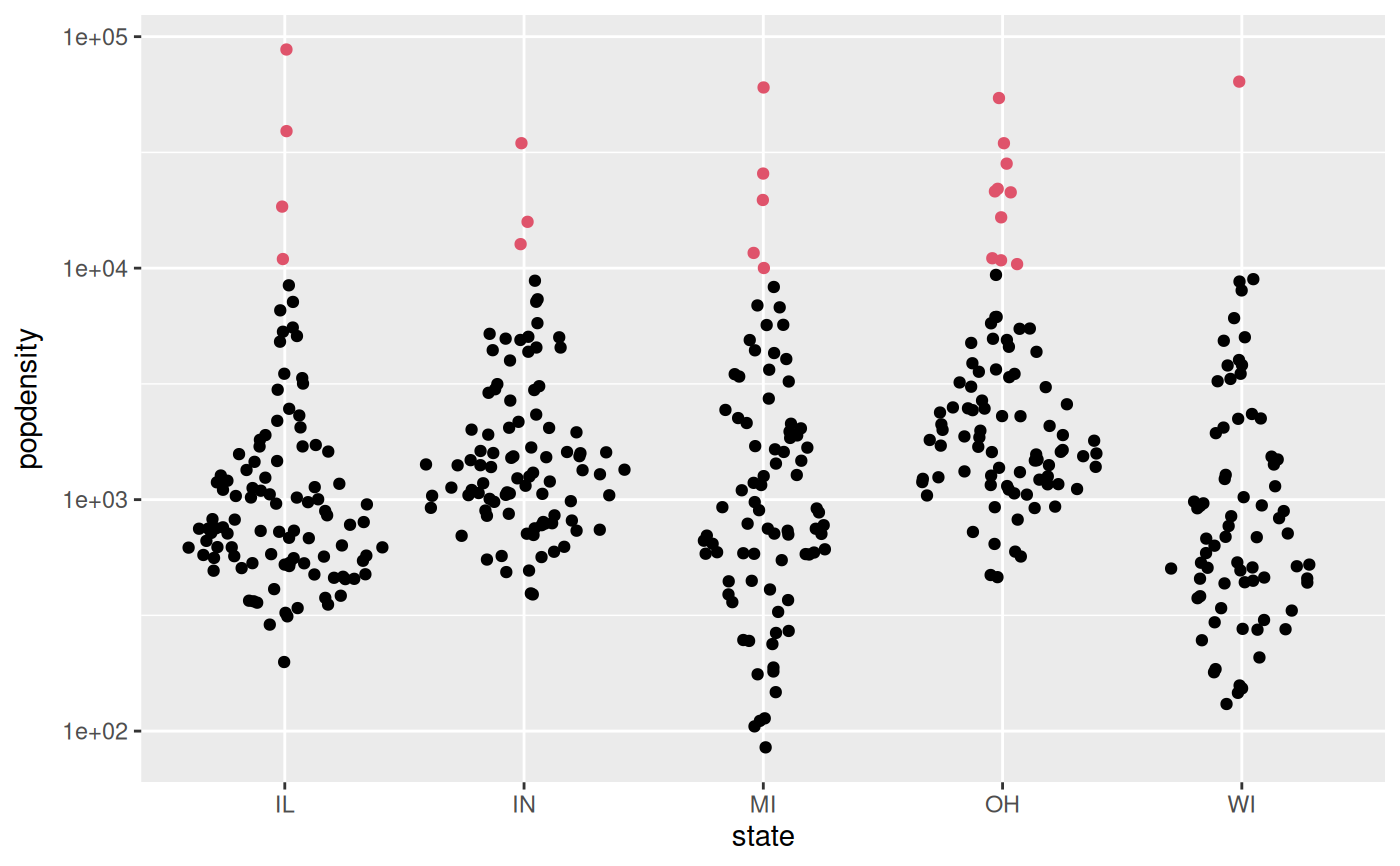# Sina plots with continuous x: ggplot(midwest, aes(cut_width(area, 0.02), popdensity)) + geom_sina() + scale_y_log10()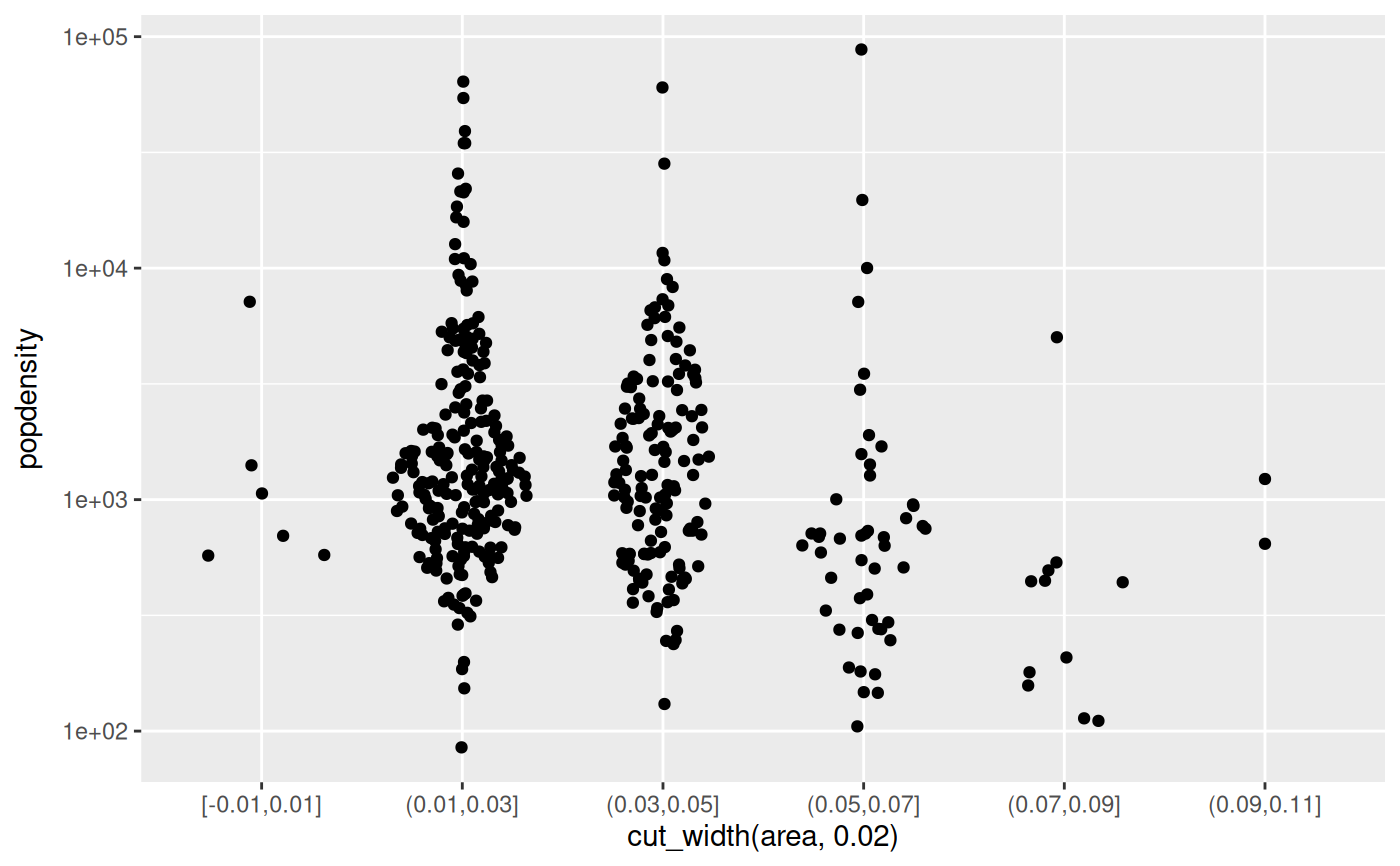### Sample gaussian distributions # Unimodal a <- rnorm(500, 6, 1) b <- rnorm(400, 5, 1.5) # Bimodal c <- c(rnorm(200, 3, .7), rnorm(50, 7, 0.4)) # Trimodal d <- c(rnorm(200, 2, 0.7), rnorm(300, 5.5, 0.4), rnorm(100, 8, 0.4)) df <- data.frame( 'Distribution' = c( rep('Unimodal 1', length(a)), rep('Unimodal 2', length(b)), rep('Bimodal', length(c)), rep('Trimodal', length(d)) ), 'Value' = c(a, b, c, d) ) # Reorder levels df$Distribution <- factor(
df$Distribution, levels(df$Distribution)[c(3, 4, 1, 2)]
)

p <- ggplot(df, aes(Distribution, Value))
p + geom_boxplot()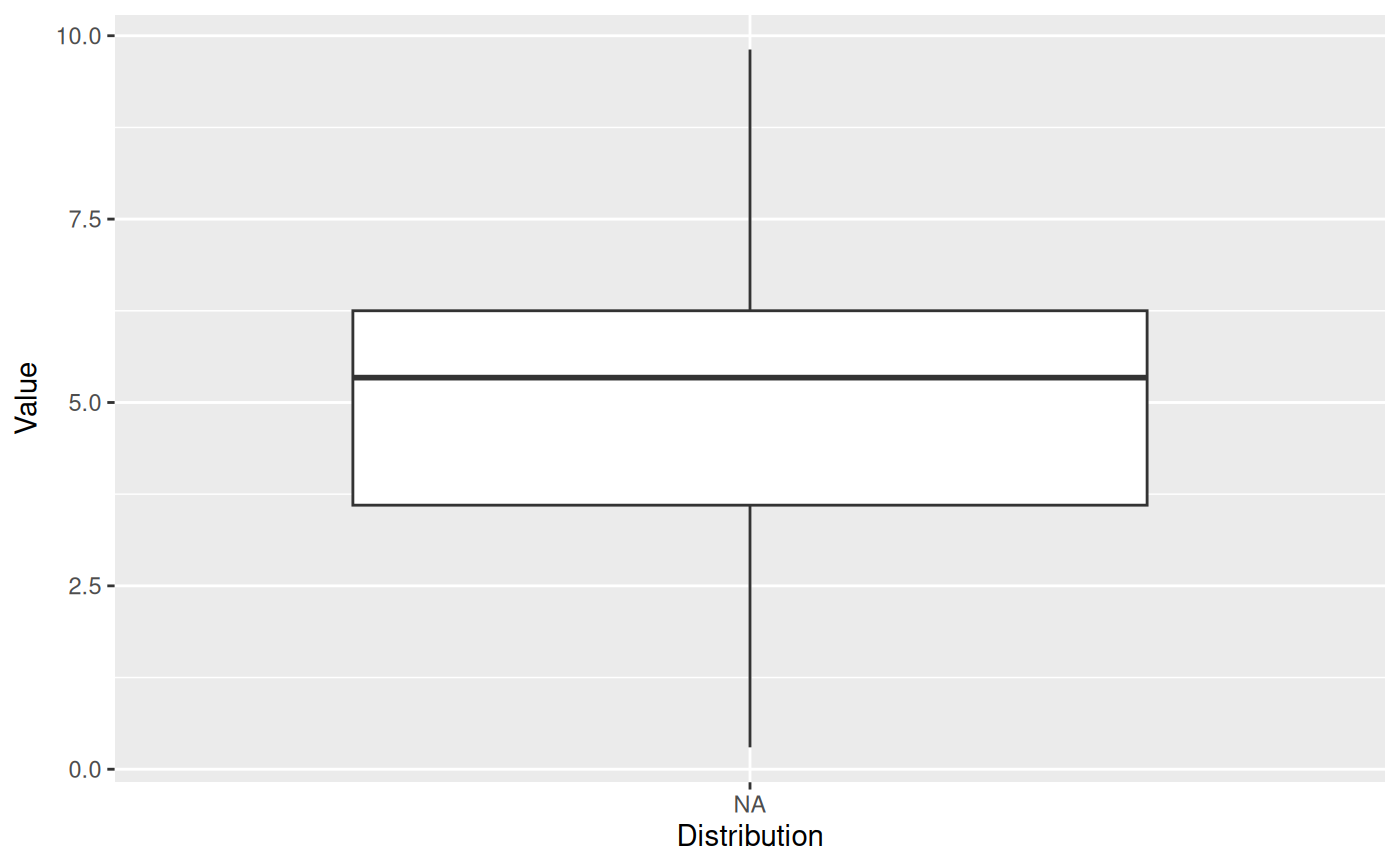p + geom_violin() +
geom_sina()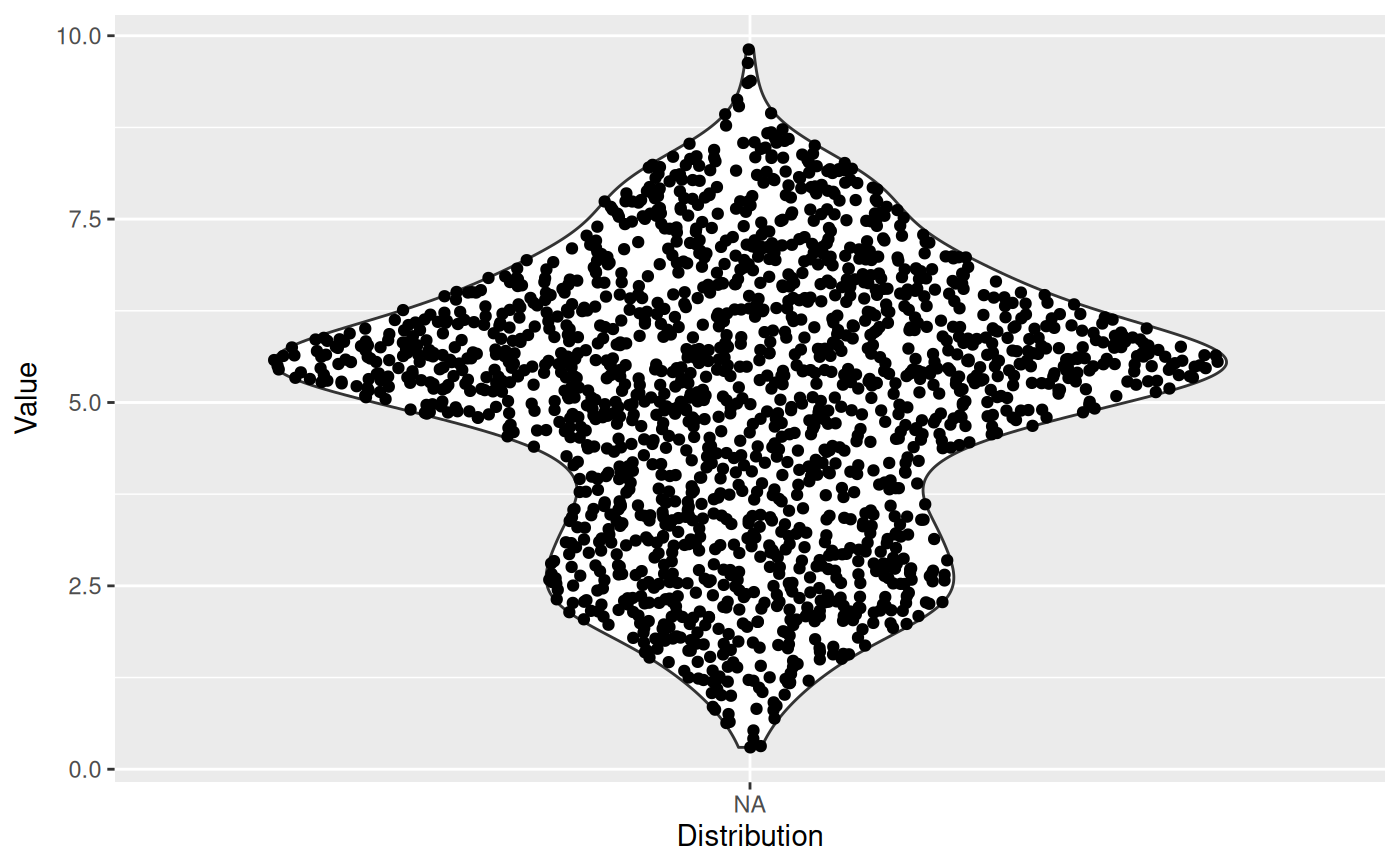# By default, Sina plot scales the width of the class according to the width
# of the class with the highest density. Turn group-wise scaling off with:
p +
geom_violin() +
geom_sina(scale = FALSE)